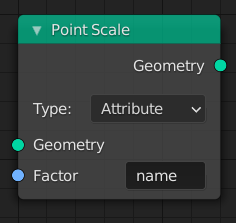# Point Scale¶The Point Scale node.

The Point Scale node changes the scale attribute of every point in the geometry by the specified amount, either from the attribute input or a vector input. This node is provided for convenience, as it’s equivalent to using the Attribute Vector Math Node with the Multiply operation and the scale attribute.

The scale attribute is used by the Point Instance Node to determine the size of every instanced object or collection.

## Inputs¶

Geometry

Standard geometry input.

Translation

The attribute or vector input.

## Properties¶

Type
Attribute

Use the values from the attribute to move each point by a different amount.

Float

Use a single value to translate every single point, this is a shortcut for assigning the same value for the X, Y, and Z, components of the vector input, and allows adjusting the scale in a uniform way.

Vector

Use a single vector to translate every single point. Equivalent to the Transform Node.

## Output¶

Geometry

Standard geometry output.